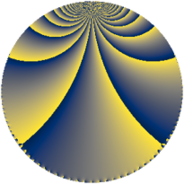# Properties

 Label 1152.2.d.dLevel $1152$ Weight $2$ Character orbit 1152.d Analytic conductor $9.199$ Analytic rank $0$ Dimension $2$ CM discriminant -4 Inner twists $4$

# Related objects

## Newspace parameters

 Level: $$N$$ $$=$$ $$1152 = 2^{7} \cdot 3^{2}$$ Weight: $$k$$ $$=$$ $$2$$ Character orbit: $$[\chi]$$ $$=$$ 1152.d (of order $$2$$, degree $$1$$, minimal)

## Newform invariants

 Self dual: no Analytic conductor: $$9.19876631285$$ Analytic rank: $$0$$ Dimension: $$2$$ Coefficient field: $$\Q(\sqrt{-1})$$ Defining polynomial: $$x^{2} + 1$$ Coefficient ring: $$\Z[a_1, \ldots, a_{5}]$$ Coefficient ring index: $$2^{2}$$ Twist minimal: no (minimal twist has level 128) Sato-Tate group: $\mathrm{U}(1)[D_{2}]$

## $q$-expansion

Coefficients of the $$q$$-expansion are expressed in terms of $$i = \sqrt{-1}$$. We also show the integral $$q$$-expansion of the trace form.

 $$f(q)$$ $$=$$ $$q + 4 i q^{5} +O(q^{10})$$ $$q + 4 i q^{5} + 4 i q^{13} + 2 q^{17} -11 q^{25} -4 i q^{29} + 12 i q^{37} -10 q^{41} -7 q^{49} + 4 i q^{53} -12 i q^{61} -16 q^{65} + 6 q^{73} + 8 i q^{85} + 10 q^{89} -18 q^{97} +O(q^{100})$$ $$\operatorname{Tr}(f)(q)$$ $$=$$ $$2q + O(q^{10})$$ $$2q + 4q^{17} - 22q^{25} - 20q^{41} - 14q^{49} - 32q^{65} + 12q^{73} + 20q^{89} - 36q^{97} + O(q^{100})$$

## Character values

We give the values of $$\chi$$ on generators for $$\left(\mathbb{Z}/1152\mathbb{Z}\right)^\times$$.

 $$n$$ $$127$$ $$641$$ $$901$$ $$\chi(n)$$ $$1$$ $$1$$ $$-1$$

## Embeddings

For each embedding $$\iota_m$$ of the coefficient field, the values $$\iota_m(a_n)$$ are shown below.

For more information on an embedded modular form you can click on its label.

Label $$\iota_m(\nu)$$ $$a_{2}$$ $$a_{3}$$ $$a_{4}$$ $$a_{5}$$ $$a_{6}$$ $$a_{7}$$ $$a_{8}$$ $$a_{9}$$ $$a_{10}$$
577.1
 − 1.00000i 1.00000i
0 0 0 4.00000i 0 0 0 0 0
577.2 0 0 0 4.00000i 0 0 0 0 0
 $$n$$: e.g. 2-40 or 990-1000 Significant digits: Format: Complex embeddings Normalized embeddings Satake parameters Satake angles

## Inner twists

Char Parity Ord Mult Type
1.a even 1 1 trivial
4.b odd 2 1 CM by $$\Q(\sqrt{-1})$$
8.b even 2 1 inner
8.d odd 2 1 inner

## Twists

By twisting character orbit
Char Parity Ord Mult Type Twist Min Dim
1.a even 1 1 trivial 1152.2.d.d 2
3.b odd 2 1 128.2.b.b 2
4.b odd 2 1 CM 1152.2.d.d 2
8.b even 2 1 inner 1152.2.d.d 2
8.d odd 2 1 inner 1152.2.d.d 2
12.b even 2 1 128.2.b.b 2
15.d odd 2 1 3200.2.d.e 2
15.e even 4 1 3200.2.f.c 2
15.e even 4 1 3200.2.f.d 2
16.e even 4 1 2304.2.a.a 1
16.e even 4 1 2304.2.a.p 1
16.f odd 4 1 2304.2.a.a 1
16.f odd 4 1 2304.2.a.p 1
24.f even 2 1 128.2.b.b 2
24.h odd 2 1 128.2.b.b 2
48.i odd 4 1 256.2.a.b 1
48.i odd 4 1 256.2.a.c 1
48.k even 4 1 256.2.a.b 1
48.k even 4 1 256.2.a.c 1
60.h even 2 1 3200.2.d.e 2
60.l odd 4 1 3200.2.f.c 2
60.l odd 4 1 3200.2.f.d 2
96.o even 8 4 1024.2.e.k 4
96.p odd 8 4 1024.2.e.k 4
120.i odd 2 1 3200.2.d.e 2
120.m even 2 1 3200.2.d.e 2
120.q odd 4 1 3200.2.f.c 2
120.q odd 4 1 3200.2.f.d 2
120.w even 4 1 3200.2.f.c 2
120.w even 4 1 3200.2.f.d 2
240.t even 4 1 6400.2.a.l 1
240.t even 4 1 6400.2.a.m 1
240.bm odd 4 1 6400.2.a.l 1
240.bm odd 4 1 6400.2.a.m 1

By twisted newform orbit
Twist Min Dim Char Parity Ord Mult Type
128.2.b.b 2 3.b odd 2 1
128.2.b.b 2 12.b even 2 1
128.2.b.b 2 24.f even 2 1
128.2.b.b 2 24.h odd 2 1
256.2.a.b 1 48.i odd 4 1
256.2.a.b 1 48.k even 4 1
256.2.a.c 1 48.i odd 4 1
256.2.a.c 1 48.k even 4 1
1024.2.e.k 4 96.o even 8 4
1024.2.e.k 4 96.p odd 8 4
1152.2.d.d 2 1.a even 1 1 trivial
1152.2.d.d 2 4.b odd 2 1 CM
1152.2.d.d 2 8.b even 2 1 inner
1152.2.d.d 2 8.d odd 2 1 inner
2304.2.a.a 1 16.e even 4 1
2304.2.a.a 1 16.f odd 4 1
2304.2.a.p 1 16.e even 4 1
2304.2.a.p 1 16.f odd 4 1
3200.2.d.e 2 15.d odd 2 1
3200.2.d.e 2 60.h even 2 1
3200.2.d.e 2 120.i odd 2 1
3200.2.d.e 2 120.m even 2 1
3200.2.f.c 2 15.e even 4 1
3200.2.f.c 2 60.l odd 4 1
3200.2.f.c 2 120.q odd 4 1
3200.2.f.c 2 120.w even 4 1
3200.2.f.d 2 15.e even 4 1
3200.2.f.d 2 60.l odd 4 1
3200.2.f.d 2 120.q odd 4 1
3200.2.f.d 2 120.w even 4 1
6400.2.a.l 1 240.t even 4 1
6400.2.a.l 1 240.bm odd 4 1
6400.2.a.m 1 240.t even 4 1
6400.2.a.m 1 240.bm odd 4 1

## Hecke kernels

This newform subspace can be constructed as the intersection of the kernels of the following linear operators acting on $$S_{2}^{\mathrm{new}}(1152, [\chi])$$:

 $$T_{5}^{2} + 16$$ $$T_{7}$$ $$T_{17} - 2$$

## Hecke characteristic polynomials

$p$ $F_p(T)$
$2$ $$T^{2}$$
$3$ $$T^{2}$$
$5$ $$16 + T^{2}$$
$7$ $$T^{2}$$
$11$ $$T^{2}$$
$13$ $$16 + T^{2}$$
$17$ $$( -2 + T )^{2}$$
$19$ $$T^{2}$$
$23$ $$T^{2}$$
$29$ $$16 + T^{2}$$
$31$ $$T^{2}$$
$37$ $$144 + T^{2}$$
$41$ $$( 10 + T )^{2}$$
$43$ $$T^{2}$$
$47$ $$T^{2}$$
$53$ $$16 + T^{2}$$
$59$ $$T^{2}$$
$61$ $$144 + T^{2}$$
$67$ $$T^{2}$$
$71$ $$T^{2}$$
$73$ $$( -6 + T )^{2}$$
$79$ $$T^{2}$$
$83$ $$T^{2}$$
$89$ $$( -10 + T )^{2}$$
$97$ $$( 18 + T )^{2}$$